• Matlab 遗传算法工具箱 基于Matlab 平台的遗传算法(GA)工具箱主要有美国北卡罗来纳大学开发 的GAOT英国谢菲尔德大学开发的GATBX 以及GADS(Genetic Algorithm and Direct Search Toolbox)遗传算法与直接搜索工具箱...
• matlab遗传算法解决背包问题，价值比为目标函数，matlab软件编程实现，没有采用工具包，按照C格式编写
• 数学建模可能用到的资源 毕业论文也可以写 使用工作箱 操作简单方便真的很方便了 老师发的资源文档
• Matlab遗传算法工具箱及应用PDF及源码，包含书籍的原文及代码，望采纳
• 这个是最好用的matlab遗传算法工具箱gatbx，该工具箱可以直接使用，带有安装说明和使用简介，文档在资源里，自行查看即可
• matlab遗传算法工具包matlab遗传算法工具包matlab遗传算法工具包
• MATLAB遗传算法系统详细讲解，学会，matlab自带的工具箱或者代码的遗传算法均有，欢迎大家来学习
• 现在上传一份MATLAB遗传算法工具箱_安装教程_使用实例），里面用两个实例很清晰地演示了如何使用此工具箱。 具体的使用过程是参考如下博文的，感谢博主的强大技术与无私奉献精神，我只是个搬运工： ...
• 如何开发多目标遗传算法。（其中附带了多目标遗传算法的程序）。以及遗传工具箱的应用！
• 一、使用单变量进行参数寻优...2、在MATLAB命令窗口输入optimtool，接下来进行选择，选择完后，点击start。 x = 1时，s取-2（最小值） 寻优曲线图如下： 二、使用双变量进行参数寻优 如s = 3x -5y ,当x,y在[...
一、使用单变量进行参数寻优
如s = -2x ,当x在[0,1]范围内，取多少时s为最小值？
使用步骤如下：
1、建立一个function函数，命名为fu.m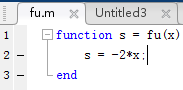2、在MATLAB命令窗口输入optimtool，接下来进行选择，选择完后，点击start。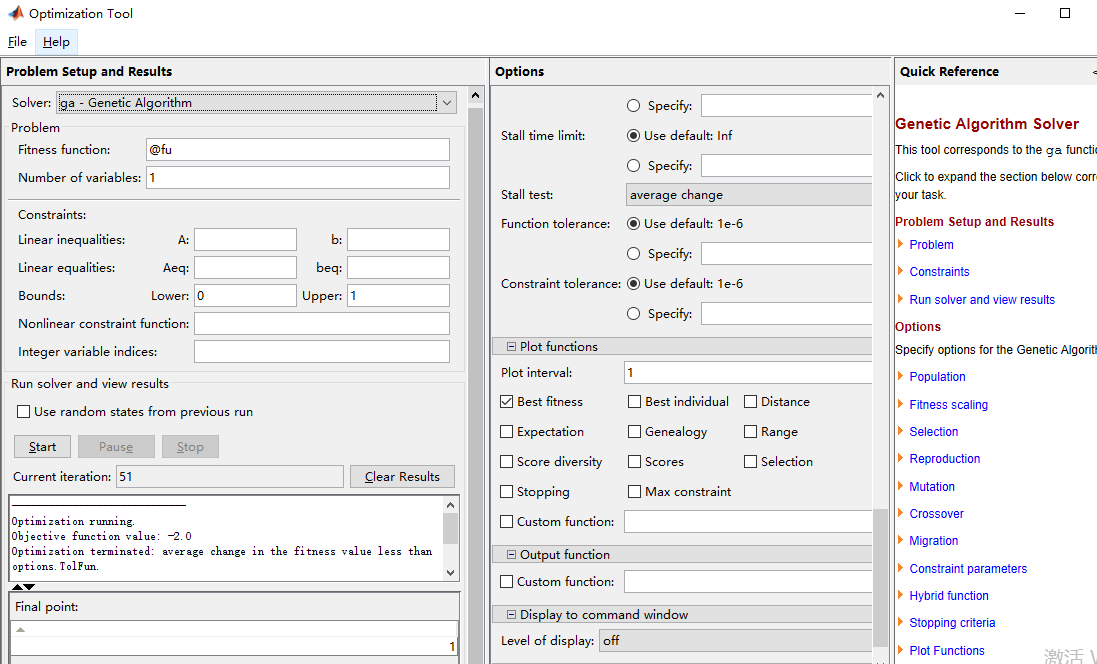x = 1时，s取-2（最小值）
寻优曲线图如下：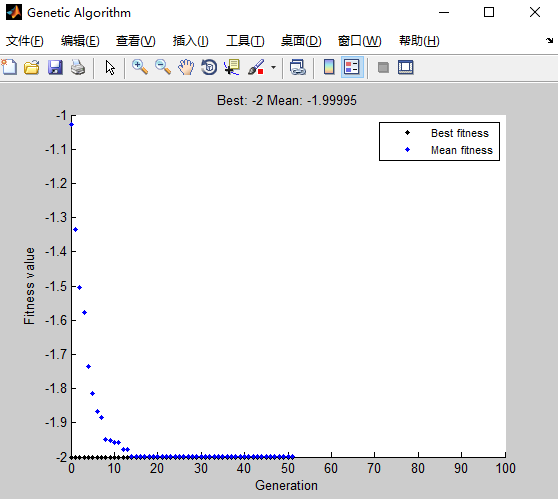二、使用双变量进行参数寻优
如s = 3x -5y ,当x,y在[0,1]范围内，取多少时s为最小值？
使用步骤如下：
1、建立一个function函数，命名为fu.m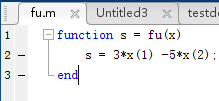2、在MATLAB命令窗口输入optimtool，接下来进行选择，选择完后，点击start。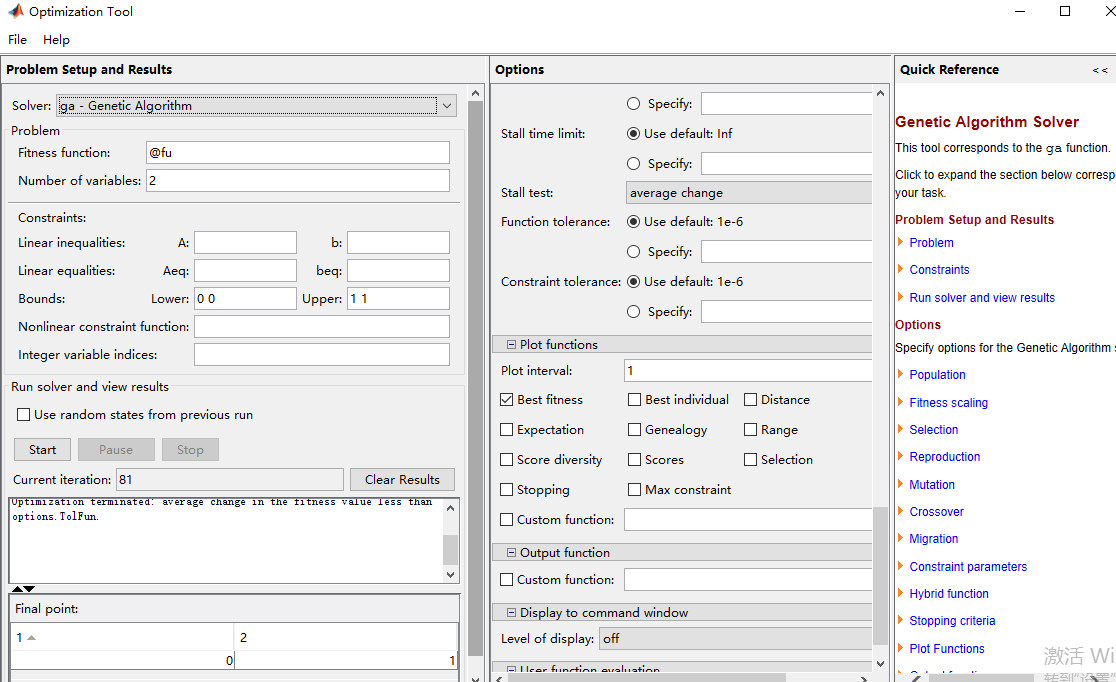x = 0, y=1时，s取-5（最小值）
寻优曲线图如下：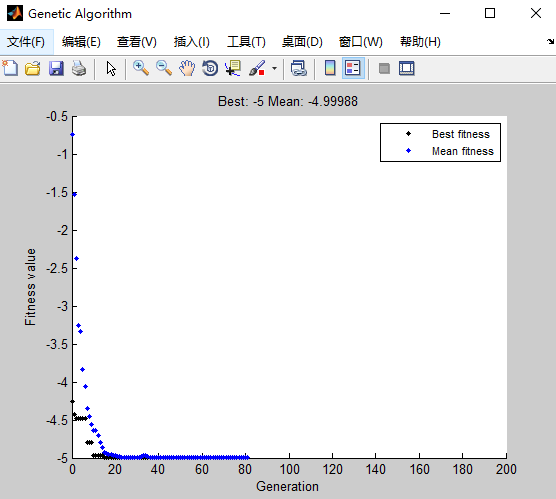PS:只是简单应用！！！

展开全文• 遗传算法概述 遗传算法（ genetic algorithm,GA）是一种进化算法，其基本原理是仿效生物界中的“物竞天择、适者生存”的演化法则。遗传算法是把问题参数编码为染色体，再利用迭代的方式进行选择、交叉以及变异等运算...
遗传算法概述
工具箱下载地址
遗传算法（ genetic algorithm,GA）是一种进化算法，其基本原理是仿效生物界中的“物竞天择、适者生存”的演化法则。遗传算法是把问题参数编码为染色体，再利用迭代的方式进行选择、交叉以及变异等运算来交换种群中染色体的信息，最终生成符合优化目标的染色体。 在遗传算法中，染色体对应的是数据或数组，通常是由一维的串结构数据来表示，串上各个位置对应基因的取值。基因组成的串就是染色体，或者称为基因型个体（ individuals）。一定数量的个体组成了群体（ population）。群体中个体的数目称为群体大小（ population size），也称为群体规模。而各个个体对环境的适应程度叫做适应度（ fitness）。 遗传算法的基本步骤如下：
1.编码
GA在进行搜索之前先将解空间的解数据表示成遗传空间的基因型串结构数据，这些串结构数据的不同组合便构成了不同的点
2.初始群体的生成
随机产生N个初始串结构数据，每个串结构数据称为一个个体，N个个体构成了一个群体。GA以这N个串结构数据作为初始点开始进化。
3.适应度评估
适应度表明个体或解的优劣性。不同的问题，适应性函数的定义方式也不同
4.选择
选择的目的是为了从当前群体中选出优良的个体，使它们有机会作为父代为下一代繁殖子孙。遗传算法通过选择过程体现这一思想，进行选择的原则是适应性强的个体为下一代贡献一个或多个后代的概率大。选择体现了达尔文的适者生存原则
5.交叉
交叉操作是遗传算法中最主要的遗传操作。通过交叉操作可以得到新一代个体，新个体组合了其父辈个体的特性。交叉体现了信息交换的思想。
6.变异
变异首先在群体中随机选择一个个体，对于选中的个体以一定的概率随机地改变串结构数据中某个串的值。同生物界一样，GA中变异发生的概率很低，通常取值很小。
工具箱添加
用户可以通过网络下载 Sheffield工具箱，然后把工具箱添加到本机的 MATLAB环境中，工具箱的安装步骤如下： （1）将工具箱文件夹复制到本地计算机中的工具箱目录下，路径为 matlabroot\ toolbox其中 matlabroot为 MATLAB的安装根目录。 （2）将工具箱所在的文件夹添加到 MATLAB的搜索路径中，有两种方式可以实现，即命令行方式和图形用户界面方式。 找到工具箱所在的文件夹（ gatbx），单击“OK”按钮，则工具箱所在的文件夹出现在 “ MATLAB search path”的最上端。单击“Save”按钮保存搜索路径的设置，然后单击“ Close” 按钮关闭对话框。 （3）查看工具箱是否安装成功。 使用函数ver查看 gatbx工具箱的名字、发行版本、发行字符串及发行日期，如果返回均为空，则说明安装未成功；如果返回了相应的参数，则表明工具箱安装成功，该工具箱就可以使用。
工具箱结构
遗传算法工具箱中的主要函数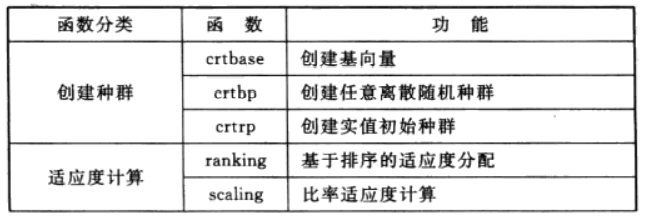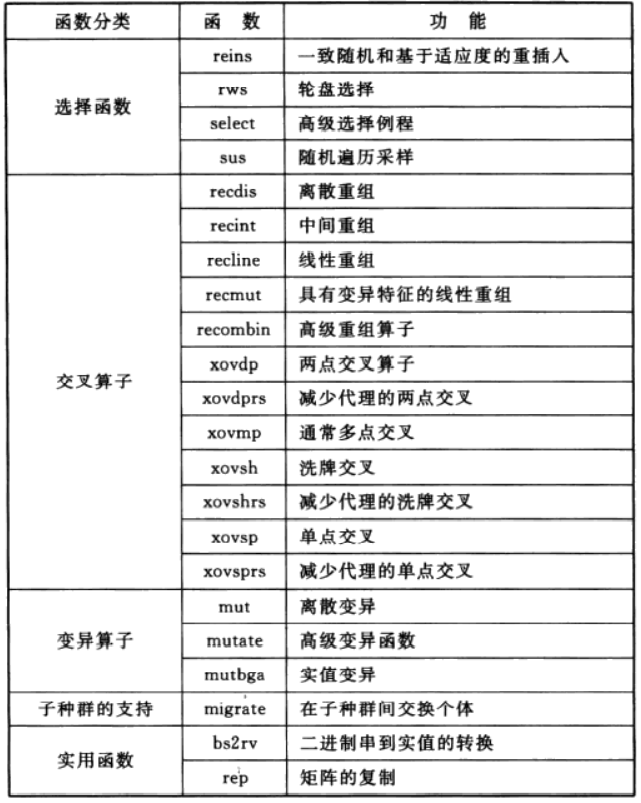遗传算法常用函数
1.创建种群函数— crbp功能：刨建任意离散随机种群。
调用格式： 1【Chrom, Lind, Basev】= crtbp（Nind, Lind） 2 【Chrom, Lind, Base v】=crtbp（Nind, Base） 3 【Chrom, Lind, Basev】= crtbp（Nind, Lind, Base） 格式①创建一个大小为Nind×Lind的随机二进制矩阵，其中，Nind为种群个体数，Lind为个体长度。返回种群编码 Chrom和染色体基因位的基本字符向量 Base v。 格式②创建一个种群个体为Nind，个体的每位编码的进制数由Base决定（Base的列数即为个体长度）。 格式③创建一个大小为Nind×Lind的随机矩阵，个体的各位的进制数由Base决定，这时输入参数Lind可省略（Base的列数即为Lind），即为格式②。 【用法举例】使用函数 crbp创建任意离散随机种群的应用举例 （1）创建一个种群大小为5，个体长度为10的二进制随机种群：
>>【Chrom, Lind, Basev】=crtbp（5,10）

（2）创建一个种群大小为5，个体长度为8，各位的进制数分别为{2,3,4,5,6,7,8,9}：
> 【Chrom, Lind, Base v】=crtbp（5， 8，【23 456789】）

2.适应度计算函数— ranking功能：基于排序的适应度分配。
调用格式： ① FitnV= ranking（0bjv （2 Fitnv= ranking（Obj, RFun） 3 Fitnv= ranking（Obj, RFun, SUBPOP格式①是按照个体的目标值ObV（列向量）由小到大的顺序对个体进行排序的，并返回个体适应度值FtnV的列向量。 格式②中RFun有三种情况： （1）若RFun是一个在【1,2】区间内的标量，则采用线性排序，这个标量指定选择的压差 （2）若RFun是一个具有两个参数的向量，则RFun（2）：指定排序方法，0为线性排序，1为非线性排序。 RFun（1）：对线性排序，标量指定的选择压差RFun（1）必须在【1,2】区间；对非线性排序，RFun（1）必须在【1， length（ObV）-2】区间；如果为NAN，则RFun（1）假设为2。 （3）若RFun是长度为 length（ObjV）的向量，则它包含对每一行的适应度值计算格式③中的参数ObjV和RFun与格式①和格式②一致，参数 SUBPOP是一个任选参数，指明在ObjⅤ中子种群的数量。省略 SUBPOP或 SUBPOP为NAN，则 SUBPOP=1.在ObV中的所有子种群大小必须相同。如果 ranking被调用于多子种群，则 ranking独立地对每个子种群执行。
3.选择函数— elect功能：从种群中选择个体（高级函数）
调用格式： 1 Selch= select（SEL F, Chrom, Fitnv） 2 Selch= select（SEL, F, Chrom, FitnV, GGAP） 3 Selch= select（SEL F, Chrom, FitnV, GGAP, SUBPOP） SELF是一个字符串，包含一个低级选择函数名，如rws或sus Fitnv是列向量，包含种群 Chrom中个体的适应度值。这个适应度值表明了每个个体被选择的预期概率。 GGAP是可选参数，指出了代沟部分种群被复制。如果GGAP省略或为NAN，则GGAP假设为1.0。 SUBPOP是一个可选参数，决定 Chron中子种群的数量。如果 SUBPOP省略或为NAN，则 SUBPOP=1。 Chrom中所有子种群必须有相同的大小
4.交叉算子函数— recombin功能：重组个体（高级函数）。
调用格式： 1 NewChrom= recombin （REC F, Chrom） 2 NewChrom= recombin（REC F, Chrom, Recopt） 3 NewChrom= recombin（REC F, Chrom, RecOpt, SUBPOP） recombin完成种群 Chrom中个体的重组，在新种群 NewChron中返回重组后的个体。 Chrom和 New Chrom中的一行对应一个个体。 RECF是一个包含低级重组函数名的字符串，例如 ecdis或 XoVsP。 RecEpt是一个指明交叉概率的任选参数，如省略或为NAN，将设为缺省值。 SUBPOP是一个决定 Chrom中子群个数的可选参数，如果省略或为NAN，则 SUBPOP为1。 Chrom中的所有子种群必须有相同的大小。
5.变异算子函数—mut功能：离散变异算子。
调用格式：Newchroa=mt（ older.m,P,BaeV Old Chrom为当前种群，Pm为变异概率（省略时为0.7/Lind）， Base指明染色体个体元素的变异的基本字符（省略时种群为二进制编码）。
6.重插入函数— . reins功能：重插入子代到种群调用格式：
1Chrom= reins（Chrom, Selch） 2 Chrom reins（Chrom, Selch, SUBPOP） 3 Chrom= reins （Chrom, SelCh, SUBPOP, InsOpt, ObjVCh） 【Chrom, Objvch】=reinsChrom, SelCh, SUBPOP, Insopt, ObjVCh, ObjVSel） reins完成插入子代到当前种群，用子代代替父代并返回结果种群。 Chron为父代种群SelCh为子代，每一行对应一个个体SUBPOP是一个可选参数，指明 Chron和SlCh中子种群的个数。如果省略或者为NAN，则假设为1.在 Chrom和SlCh中每个子种群必须具有相同大小。 InsOpt是一个最多有两个参数的任选向量。 InsOpt（1）是一个标量，指明用子代代替父代的方法。0为均匀选择，子代代替父代使用均匀随机选择。1为基于适应度的选择，子代代替父代中适应度最小的个体。如果省略Ins Opt（1）或 InsOpt（1）为NAN，则假设为0。 Ins Opt（2）是一个在【0,1】区间的标量，表示每个子种群中重插入的子代个体在整个子种群中个体的比率。如果 Ins Opt（2）省略或为NAN，则假设 Ins Opt（2）=1.0。 ObⅤCh是一个可选列向量，包括 Chrom中个体的目标值。对基于适应度的重插入， ObjvCh是必需的。 Obj vSel是一个可选参数，包含 SelCh中个体的目标值。如果子代的数量大于重插入种群中的子代数量，则 Objvsel是必需的。这种情况子代将按它们的适应度大小选择插入.
7.实用函数—bs2rv功能：二进制到十进制的转换。
调用格式：Phen=b2rv（ Chrom, Field） bs2rv根据译码矩阵 Field将二进制串矩阵 Chrom转换为实值向量，返回十进制的矩阵。
8.实用函数—rep功能：矩阵复制。
调用格式：MatOut=rep（atIn,REN函数rep完成矩阵Matn的复制，REPN指明复制次数，返回复制后的矩阵 MatOut.REPN包含每个方向复制的次数，REPN（1）表示纵向复制次数，REPN（2）表示水平方向复制次数
遣传算法工具箱应用举例
clc
clear all
close all
%% 画出函数图
figure(1);
hold on;
lb=1;ub=2; %函数自变量范围【1,2】
ezplot('sin(10*pi*X)/X',[lb,ub]);   %画出函数曲线
xlabel('自变量/X')
ylabel('函数值/Y')
%% 定义遗传算法参数
NIND=40;        %个体数目
MAXGEN=20;      %最大遗传代数
PRECI=20;       %变量的二进制位数
GGAP=0.95;      %代沟
px=0.7;         %交叉概率
pm=0.01;        %变异概率
trace=zeros(2,MAXGEN);                        %寻优结果的初始值
FieldD=[PRECI;lb;ub;1;0;1;1];                      %区域描述器
Chrom=crtbp(NIND,PRECI);                      %初始种群
%% 优化
gen=0;                                  %代计数器
X=bs2rv(Chrom,FieldD);                 %计算初始种群的十进制转换
ObjV=sin(10*pi*X)./X;        %计算目标函数值
while gen<MAXGEN
FitnV=ranking(ObjV);                               %分配适应度值
SelCh=select('sus',Chrom,FitnV,GGAP);              %选择
SelCh=recombin('xovsp',SelCh,px);                  %重组
SelCh=mut(SelCh,pm);                               %变异
X=bs2rv(SelCh,FieldD);               %子代个体的十进制转换
ObjVSel=sin(10*pi*X)./X;             %计算子代的目标函数值
[Chrom,ObjV]=reins(Chrom,SelCh,1,1,ObjV,ObjVSel); %重插入子代到父代，得到新种群
X=bs2rv(Chrom,FieldD);
gen=gen+1;                                             %代计数器增加
%获取每代的最优解及其序号，Y为最优解,I为个体的序号
[Y,I]=min(ObjV);
trace(1,gen)=X(I);                            %记下每代的最优值
trace(2,gen)=Y;                               %记下每代的最优值
end
plot(trace(1,:),trace(2,:),'bo');                            %画出每代的最优点
grid on;
plot(X,ObjV,'b*');   %画出最后一代的种群
hold off
%% 画进化图
figure(2);
plot(1:MAXGEN,trace(2,:));
grid on
xlabel('遗传代数')
ylabel('解的变化')
title('进化过程')
bestY=trace(2,end);
bestX=trace(1,end);
fprintf(['最优解:\nX=',num2str(bestX),'\nY=',num2str(bestY),'\n'])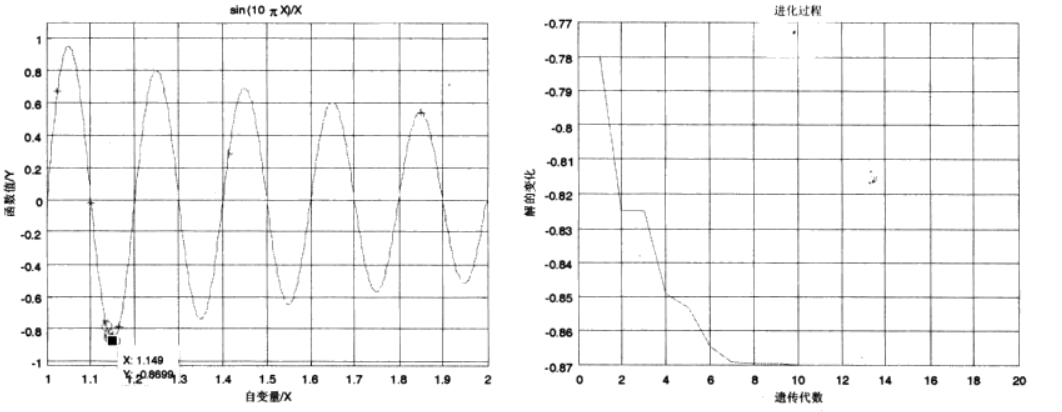展开全文• ## matlab遗传算法工具箱的使用

万次阅读 多人点赞 2018-04-15 10:35:12
前言： 1.官方文档里面有很多的实例和参数的说明 2.图形化操作之后可以...遗传算法： 在ga.m的文档中其实有很多例子 1. A = [1 1; -1 2; 2 1]; b = [2; 2; 3]; lb = zeros(2,1); % % Use mutation function wh...
前言： 1.官方文档里面有很多的实例和参数的说明 2.图形化操作之后可以自动生成代码 3.注意在程序里面要关掉打开的文件 fclose
内容提要
启用：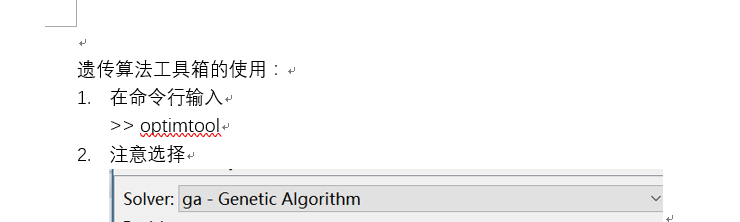遗传算法：
在ga.m的文档中其实有很多例子
1.
A = [1 1; -1 2; 2 1];  b = [2; 2; 3];  lb = zeros(2,1);
%    % Use mutation function which can handle constraints
[x,fval,exitflag] = ga(@lincontest6,2,A,b,[],[],lb,[],[],options);

2.
fun = @(x) (x(1) - 0.2)^2 + (x(2) - 1.7)^2 + (x(3) -5.1)^2;
x = ga(fun,3,[],[],[],[],[],[],[],[2 3])



3.匿名函数
x = ga(@(x) 3*sin(x(1))+exp(x(2)),2)

 4.官方文档
function [x,fval,exitFlag,output,population,scores] = ga(fun,nvars,Aineq,bineq,Aeq,beq,lb,ub,nonlcon,intcon,options)
function [解，适应度值，退出标志，输出，种群大小，得分]=ga(函数，解的维数，不等式的系数A，不等式的系数b，等式的系数A，等式的系数b)

%GA    Constrained optimization using genetic algorithm.
%   GA attempts to solve problems of the following forms:
%       min F(X)  subject to:  A*X  <= B, Aeq*X  = Beq (linear constraints)
%        X                     C(X) <= 0, Ceq(X) = 0 (nonlinear constraints)
%                              LB <= X <= UB
%                              X(i) integer, where i is in the index
%                              vector INTCON (integer constraints)
%
%   Note: If INTCON is not empty, then no equality constraints are allowed.
%   That is:-
%   * Aeq and Beq must be empty
%   * Ceq returned from NONLCON must be empty
%
%   X = GA(FITNESSFCN,NVARS) finds a local unconstrained minimum X to the
%   FITNESSFCN using GA. NVARS is the dimension (number of design
%   variables) of the FITNESSFCN. FITNESSFCN accepts a vector X of size
%   1-by-NVARS, and returns a scalar evaluated at X.
%
%   X = GA(FITNESSFCN,NVARS,A,b) finds a local minimum X to the function
%   FITNESSFCN, subject to the linear inequalities A*X <= B. Linear
%   constraints are not satisfied when the PopulationType option is set to
%   'bitString' or 'custom'. See the documentation for details.
%
%   X = GA(FITNESSFCN,NVARS,A,b,Aeq,beq) finds a local minimum X to the
%   function FITNESSFCN, subject to the linear equalities Aeq*X = beq as
%   well as A*X <= B. (Set A=[] and B=[] if no inequalities exist.) Linear
%   constraints are not satisfied when the PopulationType option is set to
%   'bitString' or 'custom'. See the documentation for details.
%
%   X = GA(FITNESSFCN,NVARS,A,b,Aeq,beq,lb,ub) defines a set of lower and
%   upper bounds on the design variables, X, so that a solution is found in
%   the range lb <= X <= ub. Use empty matrices for lb and ub if no bounds
%   exist. Set lb(i) = -Inf if X(i) is unbounded below;  set ub(i) = Inf if
%   X(i) is unbounded above. Linear constraints are not satisfied when the
%   PopulationType option is set to 'bitString' or 'custom'. See the
%   documentation for details.
%
%   X = GA(FITNESSFCN,NVARS,A,b,Aeq,beq,lb,ub,NONLCON) subjects the
%   minimization to the constraints defined in NONLCON. The function
%   NONLCON accepts X and returns the vectors C and Ceq, representing the
%   nonlinear inequalities and equalities respectively. GA minimizes
%   FITNESSFCN such that C(X)<=0 and Ceq(X)=0. (Set lb=[] and/or ub=[] if
%   no bounds exist.) Nonlinear constraints are not satisfied when the
%   PopulationType option is set to 'bitString' or 'custom'. See the
%   documentation for details.
%
%   X = GA(FITNESSFCN,NVARS,A,b,Aeq,beq,lb,ub,NONLCON,options) minimizes
%   with the default optimization parameters replaced by values in OPTIONS.
%   OPTIONS can be created with the OPTIMOPTIONS function. See OPTIMOPTIONS
%   for details. For a list of options accepted by GA refer to the
%   documentation.
%
%   X = GA(FITNESSFCN,NVARS,A,b,[],[],lb,ub,NONLCON,INTCON) requires that
%   the variables listed in INTCON take integer values. Note that GA does
%   not solve problems with integer and equality constraints. Pass empty
%   matrices for the Aeq and beq inputs if INTCON is not empty.
%
%   X = GA(FITNESSFCN,NVARS,A,b,[],[],lb,ub,NONLCON,INTCON,options)
%   minimizes with integer constraints and the default optimization
%   parameters replaced by values in OPTIONS. OPTIONS can be created with
%   the OPTIMOPTIONS function. See OPTIMOPTIONS for details.
%
%   X = GA(PROBLEM) finds the minimum for PROBLEM. PROBLEM is a structure
%   that has the following fields:
%       fitnessfcn: <Fitness function>
%            nvars: <Number of design variables>
%            Aineq: <A matrix for inequality constraints>
%            bineq: <b vector for inequality constraints>
%              Aeq: <Aeq matrix for equality constraints>
%              beq: <beq vector for equality constraints>
%               lb: <Lower bound on X>
%               ub: <Upper bound on X>
%          nonlcon: <Nonlinear constraint function>
%           intcon: <Index vector for integer variables>
%          options: <Options created with optimoptions('ga',...)>
%         rngstate: <State of the random number generator>
%
%   [X,FVAL] = GA(FITNESSFCN, ...) returns FVAL, the value of the fitness
%   function FITNESSFCN at the solution X.
%
%   [X,FVAL,EXITFLAG] = GA(FITNESSFCN, ...) returns EXITFLAG which
%   describes the exit condition of GA. Possible values of EXITFLAG and the
%   corresponding exit conditions are
%
%     1 Average change in value of the fitness function over
%        options.MaxStallGenerations generations less than
%        options.FunctionTolerance and constraint violation less than
%        options.ConstraintTolerance.
%     3 The value of the fitness function did not change in
%        options.MaxStallGenerations generations and constraint violation
%        less than options.ConstraintTolerance.
%     4 Magnitude of step smaller than machine precision and constraint
%        violation less than options.ConstraintTolerance. This exit
%        condition applies only to nonlinear constraints.
%     5 Fitness limit reached and constraint violation less than
%        options.ConstraintTolerance.
%     0 Maximum number of generations exceeded.
%    -1 Optimization terminated by the output or plot function.
%    -2 No feasible point found.
%    -4 Stall time limit exceeded.
%    -5 Time limit exceeded.
%
%   [X,FVAL,EXITFLAG,OUTPUT] = GA(FITNESSFCN, ...) returns a
%   structure OUTPUT with the following information:
%             rngstate: <State of the random number generator before GA started>
%          generations: <Total generations, excluding HybridFcn iterations>
%            funccount: <Total function evaluations>
%        maxconstraint: <Maximum constraint violation>, if any
%              message: <GA termination message>
%
%   [X,FVAL,EXITFLAG,OUTPUT,POPULATION] = GA(FITNESSFCN, ...) returns the
%   final POPULATION at termination.
%
%   [X,FVAL,EXITFLAG,OUTPUT,POPULATION,SCORES] = GA(FITNESSFCN, ...) returns
%   the SCORES of the final POPULATION.
%
%
%   Example:
%     Unconstrained minimization of 'rastriginsfcn' fitness function of
%     numberOfVariables = 2
%      x = ga(@rastriginsfcn,2)
%
%     Display plotting functions while GA minimizes
%      options = optimoptions('ga','PlotFcn',...
%        {@gaplotbestf,@gaplotbestindiv,@gaplotexpectation,@gaplotstopping});
%      [x,fval,exitflag,output] = ga(@rastriginsfcn,2,[],[],[],[],[],[],[],options)
%
%   An example with inequality constraints and lower bounds
%    A = [1 1; -1 2; 2 1];  b = [2; 2; 3];  lb = zeros(2,1);
%    % Use mutation function which can handle constraints
%    [x,fval,exitflag] = ga(@lincontest6,2,A,b,[],[],lb,[],[],options);
%
%     FITNESSFCN can also be an anonymous function:
%        x = ga(@(x) 3*sin(x(1))+exp(x(2)),2)
%
%   If FITNESSFCN or NONLCON are parameterized, you can use anonymous
%   functions to capture the problem-dependent parameters. Suppose you want
%   to minimize the fitness given in the function myfit, subject to the
%   nonlinear constraint myconstr, where these two functions are
%   parameterized by their second argument a1 and a2, respectively. Here
%   myfit and myconstr are MATLAB file functions such as
%
%        function f = myfit(x,a1)
%        f = exp(x(1))*(4*x(1)^2 + 2*x(2)^2 + 4*x(1)*x(2) + 2*x(2) + a1);
%
%   and
%
%        function [c,ceq] = myconstr(x,a2)
%        c = [1.5 + x(1)*x(2) - x(1) - x(2);
%              -x(1)*x(2) - a2];
%        % No nonlinear equality constraints:
%         ceq = [];
%
%   To optimize for specific values of a1 and a2, first assign the values
%   to these two parameters. Then create two one-argument anonymous
%   functions that capture the values of a1 and a2, and call myfit and
%   myconstr with two arguments. Finally, pass these anonymous functions to
%   GA:
%
%     a1 = 1; a2 = 10; % define parameters first
%     % Mutation function for constrained minimization
%     x = ga(@(x)myfit(x,a1),2,[],[],[],[],[],[],@(x)myconstr(x,a2),options)
%
%   Example: Solving a mixed-integer optimization problem
%   An example of optimizing a function where a subset of the variables are
%   required to be integers:
%
%   % Define the objective and call GA. Here variables x(2) and x(3) will
%   % be integer.
%   fun = @(x) (x(1) - 0.2)^2 + (x(2) - 1.7)^2 + (x(3) -5.1)^2;
%   x = ga(fun,3,[],[],[],[],[],[],[],[2 3])
%

%   Copyright 2003-2015 The MathWorks, Inc.

% If the first arg is not a gaoptimset, then it's a fitness function followed by a genome
% length. Here we make a gaoptimset from the args.


遗传算法的调用方式和参数的说明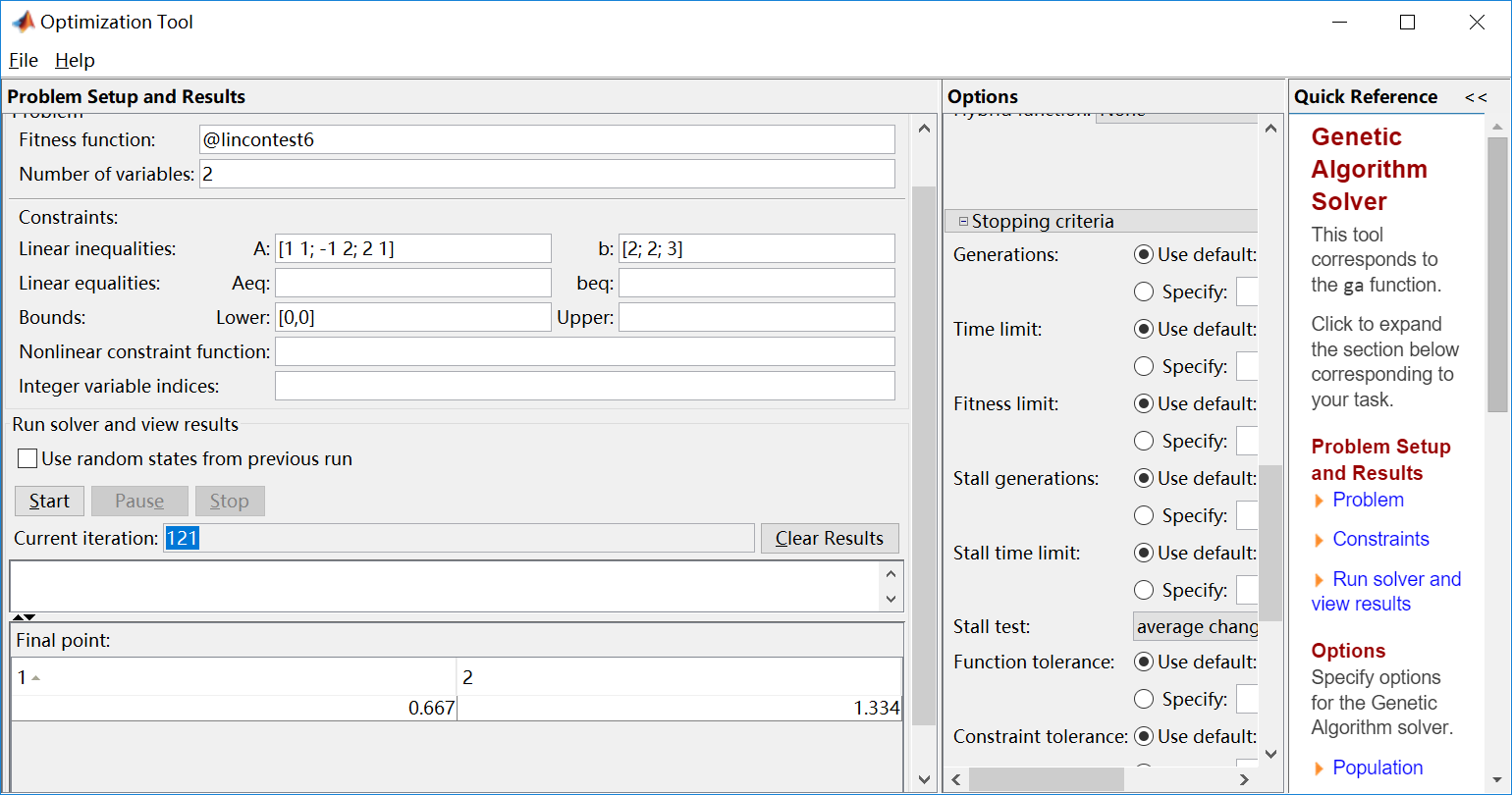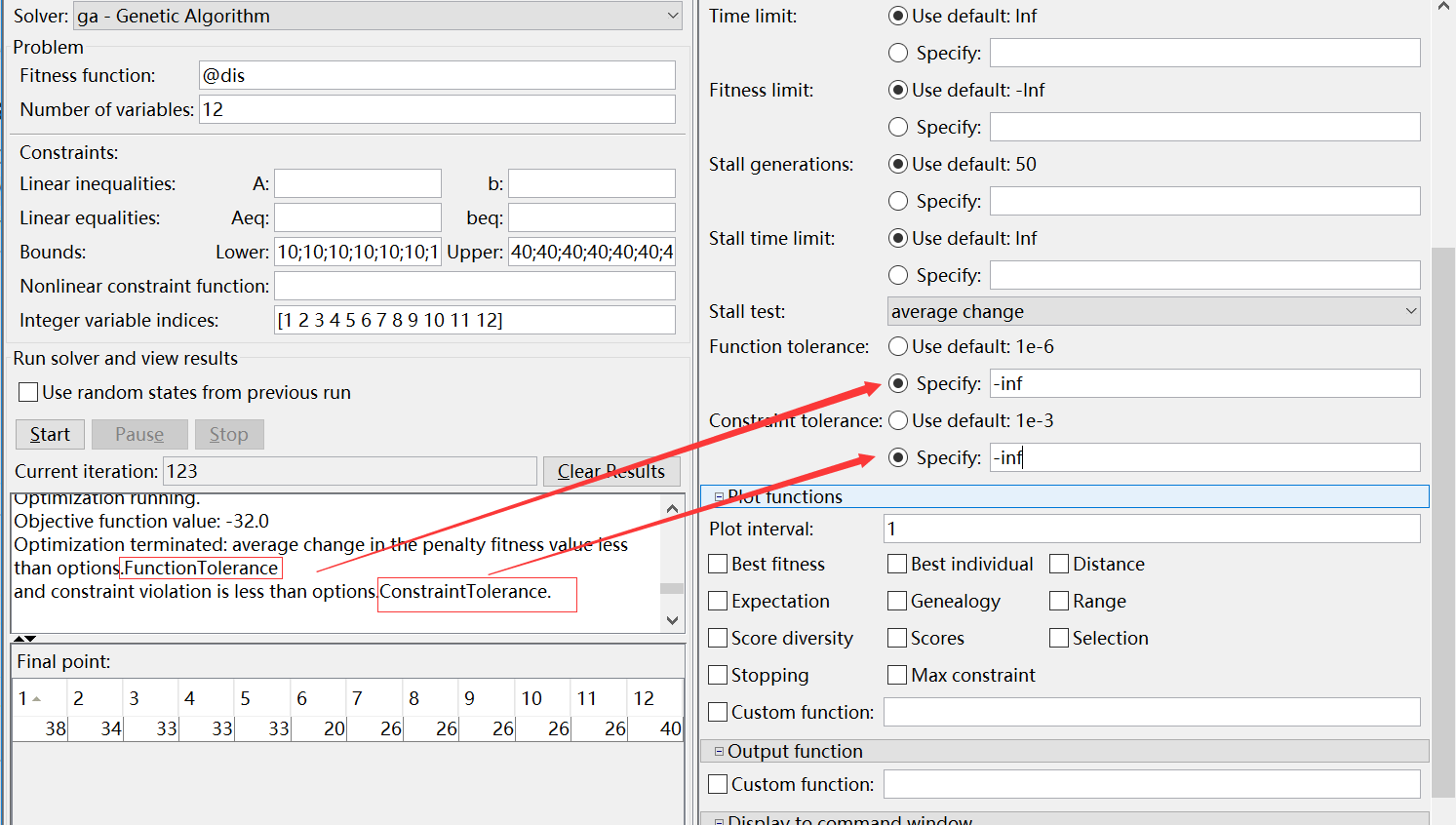可以这样查看默认参数的值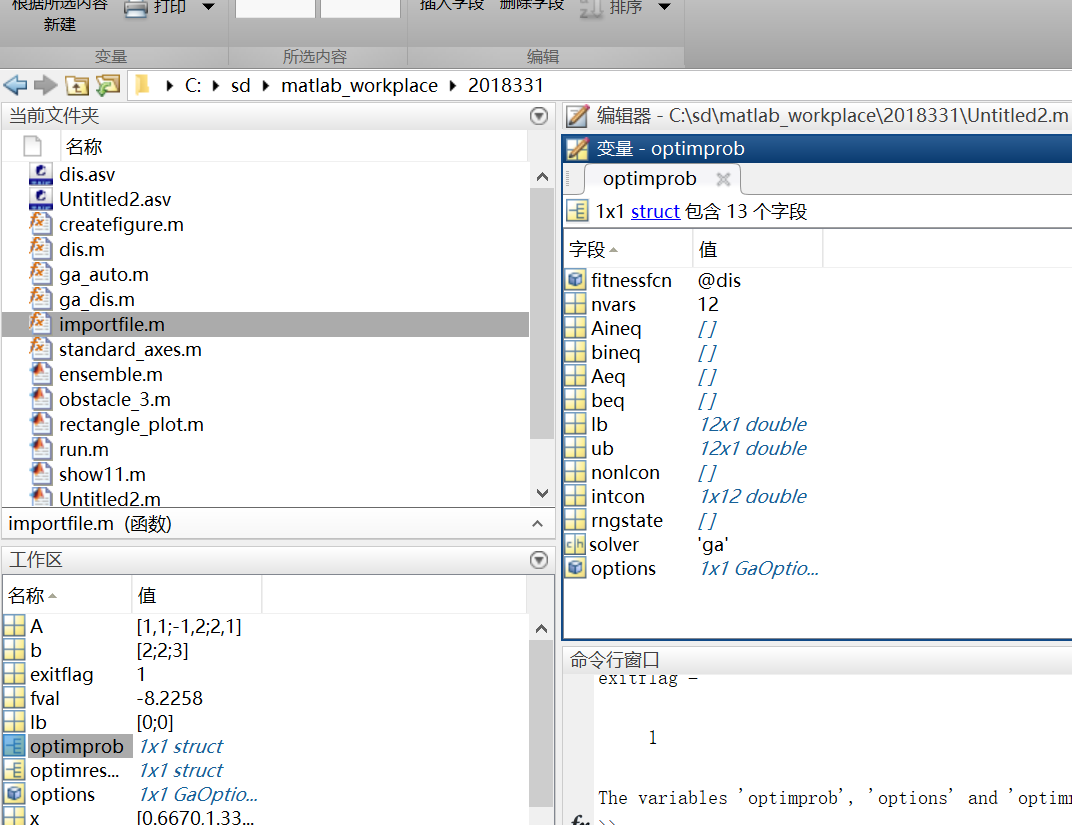A = [1 1; -1 2; 2 1];
b = [2; 2; 3];  lb = zeros(2,1);
[x,fval,exitflag] = ga(@lincontest6,2,A,b,[],[],lb)
注意顺序决定传值

遗传算法简单的几个测试用例
遗传算法测试用例
1.分段函数测试
%分段函数测试 ok
function y=ga_f_test(x)
y=0;
if x>1
y=y+x;
else
y=y+2*y;
end
end

2.关于解的测试，我觉得解应该只能是一维的

2.
A = [1 1; -1 2; 2 1];
b = [2; 2; 3];
lb = zeros(2,1);
[x,fval,exitflag] = ga(@lincontest6,2,A,b,[],[],lb)
%lb表示x的下界，up表示上界
Optimization terminated:
average change in the fitness value less than
options.TolFun.
x =     0.7794   1.2205
fval =-8.03916    exitflag =

谈谈我使用遗传算法过程中的一些坑 1.遗传算法只能生成一维的向量做为解 所以参数栏只会让你输入一个个数 2.那些参数的意义 可以见上面的 3.遗传算法适合各各样的问题，不论你的函数里面是分段的还是提前退出的，可以使用return 但是要注意的是退出的时候最好是个一个较大的随机值，而不是一个固定的值，否则最后会认为解基本上没有变化，然后就终止掉了
        if x(i)==x(j)  %一旦发现重复的值 给他一个很大的值然后马上退出
distance=randi(1000)*100000 ;

4.对于这种离散的点，其实可以先给点编号，然后随机生成1-6之间的数，再根据序号进行读值，这样可提高效率，保证有解 5.如何控制不生成重复的解目前还只能通过自己的程序进行控制
%控制一下不能有重复的值
for i=1:Number_of_trash
for j=i+1:Number_of_trash
if x(i)==x(j)  %一旦发现重复的值 给他一个很大的值然后马上退出
distance=randi(1000)*100000 ;
return ;
end
end

end


最后贴上自己的完整的代码
dis2


function distance=dis2(x)
%准备数据
%x=[1 2 3 4 5 6];
input = importfile('input.txt');
Num_of_obstacle=input{3:3,{'VarName1'}};
trash=zeros(1,3);
Number_of_trash=input{3+Num_of_obstacle+1:3+Num_of_obstacle+1,{'VarName1'}};
for i=1:Number_of_trash
trash_temp=input{3+Num_of_obstacle+1+i:3+Num_of_obstacle+1+i,{'VarName1','VarName2','VarName3'}};
trash(:,:,i)= trash_temp;
w(i)=trash_temp(1,3);
end
%把垃圾读进来
robot=input{2:2,{'VarName1','VarName2','VarName3','VarName4'}};
garbage=input{3+Num_of_obstacle+Number_of_trash+2:3+Num_of_obstacle+Number_of_trash+2,{'VarName1','VarName2'}};
w_temp=0;
weight=5;
distance=0;

%控制一下不能有重复的值
for i=1:Number_of_trash
for j=i+1:Number_of_trash
if x(i)==x(j)  %一旦发现重复的值 给他一个很大的值然后马上退出
distance=randi(1000)*100000 ;
return ;
end
end

end

for i=1:Number_of_trash
%每到达一个点 首先计算距离和权重
w_temp=w_temp+ trash(1,3,x(i));
%若没有超重
if w_temp<weight
if i==1
distance=abs(trash(1,1,x(i))-robot(1,1))+abs(trash(1,2,x(i))-robot(1,2))+distance;
else if i==Number_of_trash
distance=abs(trash(1,1,x(i))-garbage(1,1))+abs(trash(1,2,x(i))-garbage(1,2))+distance;
else if i<Number_of_trash&&i>1
distance=distance+abs(trash(1,1,x(i))-trash(1,2,x(i-1)))+abs(trash(1,1,x(i))-trash(1,1,x(i-1)));
end  %i<Number_of_trash&&i>1
end  %i==Number_of_trash
end  %i==1
else  %若是超重
%先把之前的放下，只计算当前一个的重量
w_temp=0;
w_temp=w_temp+ trash(1,3,x(i));
%这里默认第一个永远都不是超重的 所以就不会陷入死循环
%距离=到达的距离+扔垃圾的距离
if i>1
distance=abs(trash(1,1,x(i-1))-garbage(1,1))+abs(trash(1,2,x(i))-garbage(1,2))+abs(trash(1,1,x(i))-garbage(1,1))+abs(trash(1,2,x(i))-garbage(1,2))+distance;
else   %第一个垃圾就大于 weight 直接退出  一般情况下这是不可能存在的
distance=inf;
return
end
end

end


ga_dis2
function [x,fval,exitflag,output,population,score] = ga_dis2()
%% This is an auto generated MATLAB file from Optimization Tool.
nvars=6;
lb=[1 1 1 1 1 1];
ub=[6 6 6 6 6 6];
intcon=[1 2 3 4 5 6];
FunctionTolerance_Data=1e-6;
ConstraintTolerance_Data=1e-3;
options = optimoptions('ga');
%% Modify options setting
options = optimoptions(options,'FunctionTolerance', FunctionTolerance_Data);
options = optimoptions(options,'ConstraintTolerance', ConstraintTolerance_Data);
options = optimoptions(options,'Display', 'off');
[x,fval,exitflag,output,population,score] = ...
ga(@dis2,nvars,[],[],[],[],lb,ub,[],intcon,options);


展开全文• 第五章至第七章介绍英国设菲尔德（Sheffield）大学的MATLAB遗传算法工具箱及其使用方法，举例说明如何利用遗传算法工具箱函数编写求解实际优化问题的MATLAB程序。第八章和第九章介绍MathWorks公司最新发布的MATLAB...
• 1. 下载工具包。Shefield开发的工具包2. 解压。解压后，你应该得到一个genetic文件夹，如果不是，重命名为genetic。3. 复制到Matlab路径下复制文件夹genetic 到C:\program files\MATLAB\r2010a\toolbox r2010a是...
1. 下载工具包。
Shefield开发的工具包
2. 解压。解压后，你应该得到一个genetic文件夹，如果不是，重命名为genetic。
3. 复制到Matlab路径下
复制文件夹genetic 到C:\program files\MATLAB\r2010a\toolbox r2010a是版本号，找你自己的版本号。如果你的MATLAB不是装在C盘，找你自己的路径。
4.  将具箱路径加入Matlab文件路径之中  运行MATLAB, File-->Set Path-->Add Folder，选择C:\program files\MATLAB\R2010a\toolbox\ genetic文件夹加入该路径系统中。如果你不能加载路径，试试用管理员身份运行matlab。重启MATLAB。注意，你在Sheffield大学网页上下载到的GA工具箱是他们为MATLAB4.2版本编写的，它也可以用于MATLAB后面所有的更高级的版本。

CSDN上有一个这样的工具包也可以下载。然而文件名是大写的。而matlab又是区分大小写的……

我再重新上传一个把文件名都改成了小写的压缩包。

展开全文算法
• 本文将使用到Sheffield遗传算法工具箱。Sheffield遗传算法工具箱是由英国谢菲尔德大学开发的。该工具箱提供了大量的遗传算法应用到的m函数，为我们研究遗传算法提供了非常多的便捷。 我将在下面通过一个实例来介绍...
• 可下载MATLAB的gatbx安装包 1.下载后，解压。 2.将解压后的gatbx文件夹移到MATLAB的安装目录下的toolbox文件夹里 例如：D:\matlab\toolbox 3.选择 设置路径 4.添加文件夹 5.选择“预设路径”上方的...安装软件
• 本算法是用matlab求解函数极值，程序的框架是遗传算法的框架，解决不同的问题时，稍微修改一下就行了，基本的框架是不变的。包含了源程序，算法说明，包括一份详细得报告，读者应该能比较容易的看懂。
• Matlab遗传算法工具箱的使用   遗传算法（Genetic Algorithm，GA）是J.Holland教授1975年在其专著中首次发表，又经Goldberg、DeJong等研究总结形成的一种模拟进化算法，其基于生物进化和物种选择理论模拟自然界...
• 本文将介绍MATLAB遗传算法工具箱求解非线性规划问题。在阅读本文之前，建议读者阅读上一期“MATLAB遗传算法工具箱求解线性规划问题”。文章传送门：...非线性规划 数学建模
• 这样的公式，用常规的方法比较难以求解和的关系，可以采用Matlab自带的优化工具遗传算法模块进行快速求解近似关系。 （1）首先转化公式： 首先将公式转化成优化目标和约束的关系式，如下： min s.t. 进一步...
• Matlab遗传算法工具箱的使用及实例(非线性规划) 本文将介绍MATLAB遗传算法工具箱求解非线性规划问题。在阅读本文之前，建议读者阅读上一期“MATLAB遗传算法工具箱求解线性规划问题”。文章传送门： Matlab遗传算法...
• 第五章至第七章介绍英国设菲尔德（Sheffield）大学的MATLAB遗传算法工具箱及其使用方法，举例说明如何利用遗传算法工具箱函数编写求解实际优化问题的MATLAB程序。第八章和第九章介绍MathWorks公司最新发布的MATLAB...
• matlab中的扩展工具箱GAOT，做遗传算法的必备工具箱，matlab自身并不携带。下载之后，解压放在matlab的安装目录下的toolbox中，然后在matlab的主页，选择‘设置路径’》添加并包含子文件夹，选择刚才那个解压的文件...
• 基于Python语音开发的遗传算法工具箱，能够快速实现更各类参数优化等功能，并提高性能。
• matlab遗传算法工具箱（包含指导书），书中介绍了遗传算法及其改进和对应的matlab函数工具包及应用
• 里面包含了所有需要的遗传算法工具包，可以内置到matlab中，也有指导手册
• 第八章 使用 MATLAB 遗传算法工具 最新发布的 MA TLAB 7.0 Release 14 已经包含了一个专门设计的遗传算法与直接搜索工具 箱 Genetic Algorithm and Direct Search Toolbox GADS 使用遗传算法与直接搜索工具箱 可以...
• 谢菲尔德(Sheffield)遗传算法工具箱(Matlab_toolbox)新版(.m小写文件后缀)...

# matlab遗传算法工具包matlab 订阅